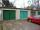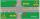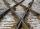# Pythagorean theorem + unit conversion - math problems

#### Number of problems found: 46

• Is right triangleFind out if the triangle ABC (with right angle at the vertex C) is right if: a) a = 3dm, b = 40cm, c = 0.5m b) a = 8dm, b = 1.2m, c = 6dm
• SailboatThe 20 m long sailboat has an 8 m high mast in the middle of the deck. The top of the mast is fixed to the bow and stern with a steel cable. Determine how much cable is needed to secure the mast and what angle the cable will make with the ship's deck.
• Cable carFind the elevation difference of the cable car when it rises by 67 per mille and the rope length is 930 m.
• Isosceles trapezoidThe old father decided to change the top plate of an isosceles-like trapezoid with the basic dimensions of 120 cm and 60 cm, and the shoulder is 50 centimeters long. How much does it pay for a new plate and a square meter worth 17 euros?
• SquareCalculate the area of the square shape of the isosceles triangle with the arms 50m and the base 60m. How many tiles are used to pave the square if the area of one tile is 25 dm2?From the junction of two streets that are perpendicular to each other, two cyclists (each on another street) walked out. One ran 18 km/h and the second 24 km/h. How are they away from a) 6 minutes, b) 15 minutes?
• DecagonCalculate the area and circumference of the regular decagon when its radius of a circle circumscribing is R = 1m
• Right angled triangle 3Side b = 1.5, hypotenuse angle A = 70 degrees, Angle B = 20 degrees. Find its unknown sides length.
• LathsThere are two laths in the garage opposite one another: one 2 meters long and the other 3 meters long. They fall against each other and lean against the opposite walls of the garage both laths and touch at a height of 70 cm above the garage floor. How wid
• Two cyclistsTwo cyclists started from crossing in the same time. One goes to the north speed 20 km/h, the second eastward at speed 26 km/h. What will be the direct distance cycling 30 minutes from the start?
• Two aircraftTwo planes fly to the airport. At some point, the first airplane is away from the airport 98 km and the second 138 km. The first aircraft flies at an average speed of 420 km/h, the second average speed is 360 km/h, while the tracks of both planes are perp
• Dig water wellMr. Zeman digging a well. Its diameter is 120 cm and plans to 3.5 meters deep. How long (at least) must be a ladder, after which Mr. Zeman would have eventually come out?
• JourneyCharles and Eva stands in front of his house, Charles went to school south at speed 5.4 km/h, Eva went to the store on a bicycle eastwards at speed 21.6 km/h. How far apart they are after 10 minutes?
• Right trapezoidThe right trapezoid has bases 3.2 cm and 62 mm long. The shorter leg has a length of 0.25 dm. Calculate the lengths of the diagonals and the second leg.
• RectangleThe rectangle is 21 cm long and 38 cm wide. Determine the radius of the circle circumscribing rectangle.
• MovementFrom the crossing of two perpendicular roads started two cyclists (each at the different road). One runs at average speed 28 km/h, the second at average speed 24 km/h. Determine the distance between them after 45 minutes cycling.
• Gimli GliderAircraft Boeing 767 lose both engines at 42000 feet. The plane captain maintain optimum gliding conditions. Every minute, lose 1910 feet and maintain constant speed 211 knots. Calculate how long it takes to plane from engine failure to hit the ground. Cal
• Track arcTwo straight tracks is in an angle 74°. They will join with circular arc with radius r=1127 m. How long will be arc connecting these lines (L)? How far is the center point of arc from track crossings (x)?
• Widescreen monitorComputer businesses hit by a wave of widescreen monitors and televisions. Calculate the area of ​​the LCD monitor with a diagonal size 20 inches at ratio 4:3 and then 16:9 aspect ratio. Is buying widescreen monitors with the same diagonal more advantageou
• Glass mosaicHow many dm2 glass is nessesary to produc 97 slides of a regular 6-gon, whose side has length 21 cm? Assume that cutting glass waste is 10%.

Do you have an exciting math question or word problem that you can't solve? Ask a question or post a math problem, and we can try to solve it.

We will send a solution to your e-mail address. Solved examples are also published here. Please enter the e-mail correctly and check whether you don't have a full mailbox.

Pythagorean theorem is the base for the right triangle calculator. Pythagorean theorem - math problems. Unit conversion - math problems.